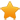Switch Editions?
CancelChannel: CodeSection,代码区,网络安全 - CodeSec
Viewing all articles

# Automated Dashboard with various correlation visualizations in R

0
0

(This article was first published on R Programming DataScience+ , and kindly contributed toR-bloggers)

Categories

Programming

Tags

Correlation Data Visualisation R Programming

In this article, you learn how to make Automated Dashboard with various correlation visualizations in R. First you need to install the `rmarkdown` package into your R library. Assuming that you installed the `rmarkdown`, next you create a new `rmarkdown` script in R.

After this you type the following code in order to create a dashboard with rmarkdown and flexdashboard :

--- title: "Dashboard visualizations in R: Scatter plots" author: "Kristian Larsen" output: flexdashboard::flex_dashboard: orientation: rows vertical_layout: scroll --- ```{r setup, include=FALSE} library(flexdashboard) # install.packages("ggplot2") # load package and data options(scipen=999) # turn-off scientific notation like 1e+48 library(ggplot2) theme_set(theme_bw()) # pre-set the bw theme. data("midwest", package = "ggplot2") midwest <- read.csv("http://goo.gl/G1K41K") # bkup data source options(scipen = 999) library(ggplot2) library(ggalt) library(plotly) midwest_select 350000 & midwest\$poptotal 0.01 & midwest\$area < 0.1, ] # load package and data library(ggplot2) data(mpg, package="ggplot2") # alternate source: "http://goo.gl/uEeRGu") theme_set(theme_bw()) # pre-set the bw theme. g <- ggplot(mpg, aes(cty, hwy)) # load package and data library(ggplot2) data(mpg, package="ggplot2") mpg <- read.csv("http://goo.gl/uEeRGu") # load package and data library(ggplot2) data(mpg, package="ggplot2") # mpg <- read.csv("http://goo.gl/uEeRGu") ``` Row ----------------------------------------------------------------------- ### Chart A: Scatterplot ```{r} gg <- ggplot(midwest, aes(x=area, y=poptotal)) + geom_point(aes(col=state, size=popdensity)) + geom_smooth(method="loess", se=F) + xlim(c(0, 0.1)) + ylim(c(0, 500000)) + labs(subtitle="Area Vs Population", y="Population", x="Area", title="Scatterplot", caption = "Source: midwest") plot(gg) ggplotly(p = ggplot2::last_plot()) ``` ### Chart B: Scatterplot + Encircle ```{r} ggplot(midwest, aes(x=area, y=poptotal)) + geom_point(aes(col=state, size=popdensity)) + # draw points geom_smooth(method="loess", se=F) + xlim(c(0, 0.1)) + ylim(c(0, 500000)) + # draw smoothing line geom_encircle(aes(x=area, y=poptotal), data=midwest_select, color="red", size=2, expand=0.08) + # encircle labs(subtitle="Area Vs Population", y="Population", x="Area", title="Scatterplot + Encircle", caption="Source: midwest") ``` Row ----------------------------------------------------------------------- ### Cart C: Jitter Plot ```{r} g + geom_point() + geom_smooth(method="lm", se=F) + labs(subtitle="mpg: city vs highway mileage", y="hwy", x="cty", title="Scatterplot with overlapping points", caption="Source: midwest") ggplotly(p = ggplot2::last_plot()) ``` ### Cart D: Jitter Points ```{r} # Scatterplot theme_set(theme_bw()) # pre-set the bw theme. g <- ggplot(mpg, aes(cty, hwy)) g + geom_jitter(width = .5, size=1) + labs(subtitle="mpg: city vs highway mileage", y="hwy", x="cty", title="Jittered Points") ggplotly(p = ggplot2::last_plot()) ``` Row ----------------------------------------------------------------------- ### Chart E: Counts Chart ```{r} # Scatterplot theme_set(theme_bw()) # pre-set the bw theme. g <- ggplot(mpg, aes(cty, hwy)) g + geom_count(col="tomato3", show.legend=F) + labs(subtitle="mpg: city vs highway mileage", y="hwy", x="cty", title="Counts Plot") ggplotly(p = ggplot2::last_plot()) ``` ### Chart F: Bubble plot ```{r} # load package and data library(ggplot2) library(gganimate) data(mpg, package="ggplot2") # mpg <- read.csv("http://goo.gl/uEeRGu") mpg_select <- mpg[mpg\$manufacturer %in% c("audi", "ford", "honda", "hyundai"), ] # Scatterplot theme_set(theme_bw()) # pre-set the bw theme. g <- ggplot(mpg_select, aes(displ, cty)) + labs(subtitle="mpg: Displacement vs City Mileage", title="Bubble chart") g + geom_jitter(aes(col=manufacturer, size=hwy)) + geom_smooth(aes(col=manufacturer), method="lm", se=F) ggplotly(p = ggplot2::last_plot()) ``` Row ----------------------------------------------------------------------- ### Chart G: Marginal Histogram / Boxplot ```{r} # load package and data library(ggplot2) library(ggExtra) data(mpg, package="ggplot2") # mpg <- read.csv("http://goo.gl/uEeRGu") # Scatterplot theme_set(theme_bw()) # pre-set the bw theme. mpg_select = 35 & mpg\$cty > 27, ] g <- ggplot(mpg, aes(cty, hwy)) + geom_count() + geom_smooth(method="lm", se=F) ggMarginal(g, type = "histogram", fill="transparent") ggMarginal(g, type = "boxplot", fill="transparent") # ggMarginal(g, type = "density", fill="transparent") ``` ### Chart H: Correlogram ```{r} # devtools::install_github("kassambara/ggcorrplot") library(ggplot2) library(ggcorrplot) # Correlation matrix data(mtcars) corr <- round(cor(mtcars), 1) # Plot ggcorrplot(corr, hc.order = TRUE, type = "lower", lab = TRUE, lab_size = 3, method="circle", colors = c("tomato2", "white", "springgreen3"), title="Correlogram of mtcars", ggtheme=theme_bw) ```

Screenshot:

The result of the above coding are published with RPubs here .

References Using flexdashboard in R

Related Post

Automated Dashboard with Visualization and Regression for Healthcare Data Create easy automated dashbords with R and Markdown Send Desktop Notifications from R in windows, linux and Mac CHAID vs. ranger vs. xgboost ― a comparison Common Mistakes to Avoid When Learning to Code in python

Viewing all articles